# Siblings

Three siblings had saved up a total of 1,274 CZK. Peter had saved up to 15% more than Jirka and Hanka 10% less than Peter. How much money did they save each one of them?

h =  414
j =  400
p =  460

### Step-by-step explanation:

p+h+j=1274
p = 1.15 j
h = 0.90 p

p+h+j=1274
p = 1.15•j
h = 0.90•p

h+j+p = 1274
1.15j-p = 0
h-0.9p = 0

h = 414
j = 400
p = 460

Our linear equations calculator calculates it.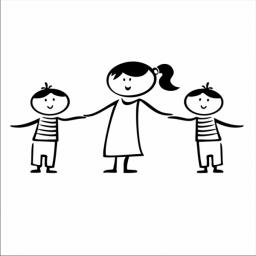Did you find an error or inaccuracy? Feel free to write us. Thank you!Tips to related online calculators
Do you have a system of equations and looking for calculator system of linear equations?

## Related math problems and questions:

• Czech coins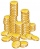John has 540 CZK, but he has 10 CZK coins and 20 CZK coins only. We know that he has 10CZK coins 7-times more than 20CZK coins. How many 10CZK coins and how many 20CZK coins did he save?
• RemunerationTotal remuneration for workers is 47 000 CZK. The second worker received 20% more than the first and third 4000 CZK less than a second employee. How many CZK each received?
• Sisters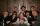Sisters Janka and Danka are saved together 220 CZK. On a trip to Janka wants to take a fifth of her savings and Danka's quarter. Then they will have 50 CZK on the trip. How much money saved Janka and Danka?
• RepairingThree employees earned a total of € 469 for repairing the equipment. They split so that the first got 20% more than the second, and the third 15% more than the second. How many euros did everyone get?
• The TV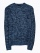The TV costs CZK 9,999. First, they reduced her price by 15%, then they became more expensive by 15%. How much does television cost now?
• Luggage and air travelTwo friends traveling by plane had a total of 35 kg of luggage. They paid one 72 CZK and the second 108 CZK for being overweight. If only one paid for all the bags, it would cost 300 CZK. What weight of baggage did each of them have? How many kilograms of
• Four pupilsFour pupils divided \$ 1485 so that the second received 50% less than the first, the third 1/2 less than a fourth, and fourth \$ 154 less than the first. How much money had each of them?
• Coins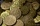The boy collected coins with value 5 CZK and 2 CZK when he had 50 pieces saved 190 CZK. How many he has each type of coin?
• RewardThree workers shared a common reward 13110 CZK: the first worker got 35% less than the second, and the third worker got 20% more than the second worker. How much got each worker?
• Crowns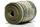1180 crowns are divided into three people: A got 20% less than B and C by 15% more than B. How many got A, B, and C?
• ContestantsThe three best contestants are to divide the total prize of CZK 4,200. The second gets 20% more than the third. And the first one gets 200 CZK less than the second and the third together. How much will everyone get?
• Dividing moneyThomas, Honza and Vasek are to divide 1220kč. Honza got 25% more than Thomas. Vasek 20% less than Thomas. How CZK each got?
• 3 masonsThree masons received 7,700 CZK. The second half received 1/2 more than the first and third twice more than the second mason. How much they each got crowns?
• Eva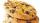When Eva buys 8 packages of cookies, 4 CZK left her. If she wanted to buy 10 packages, she would have to borrow 20 CZK. How much money has Eva in her wallet?
• Energy savingThey were released three different, independent inventions saving 20%, 24% and 15% energy. Some considered that while the use of these inventions, the total savings will be 20% + 24% + 15% = 59%. Is this true? How much will percent of energy save all thre
• Part-time workersThree part-time workers received CZK 1,235 for their work. The first received 20% less than the second and the third received 45 CZK more than the second. Determine how many crowns (CZK) each of them received.
• Two teas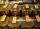A mixture weighing 10 kg was made from two types of tea. The price of tea was 160 CZK/kg and second tea 170 CZK/kg. The price of the mixture is 166 CZK/kg. How many kilograms of each type of tea had to be mixed?